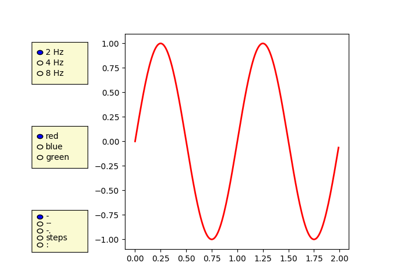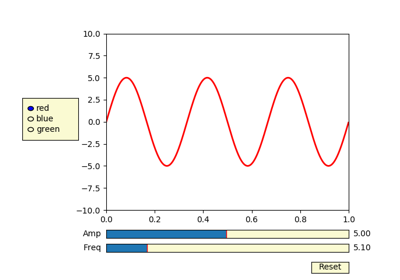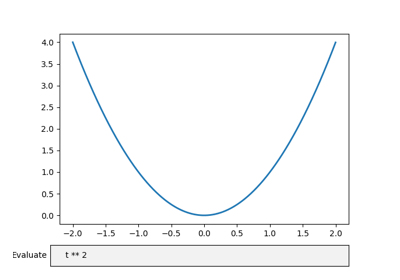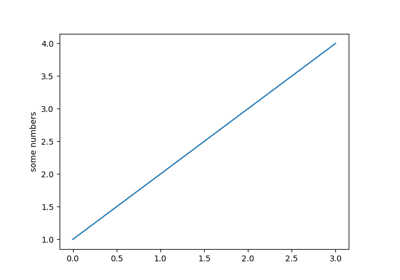You are reading an old version of the documentation (v3.0.0). For the latest version see https://matplotlib.org/stable/api/_as_gen/matplotlib.pyplot.subplots_adjust.html

### Related Topics

`matplotlib.pyplot.``subplots_adjust`(left=None, bottom=None, right=None, top=None, wspace=None, hspace=None)[source]

Tune the subplot layout.

The parameter meanings (and suggested defaults) are:

```left  = 0.125  # the left side of the subplots of the figure
right = 0.9    # the right side of the subplots of the figure
bottom = 0.1   # the bottom of the subplots of the figure
top = 0.9      # the top of the subplots of the figure
wspace = 0.2   # the amount of width reserved for space between subplots,
# expressed as a fraction of the average axis width
hspace = 0.2   # the amount of height reserved for space between subplots,
# expressed as a fraction of the average axis height
```

The actual defaults are controlled by the rc file

## Examples using `matplotlib.pyplot.subplots_adjust`¶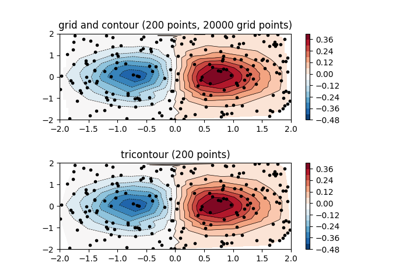Contour plot of irregularly spaced data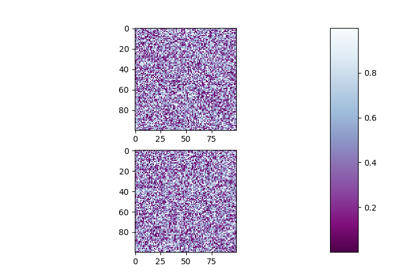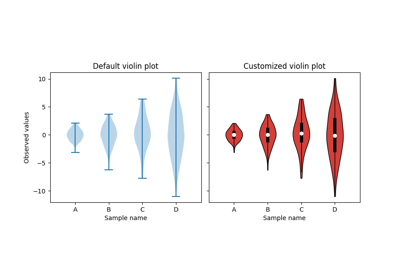Violin plot customization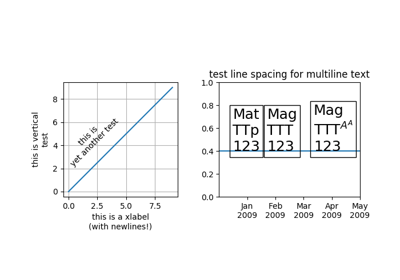Multiline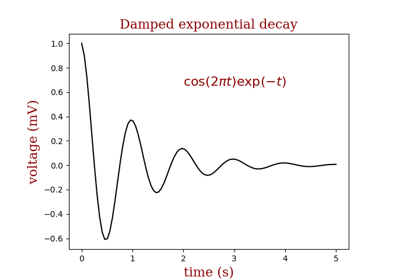Controlling style of text and labels using a dictionary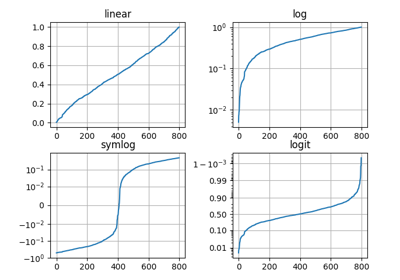Pyplot Scales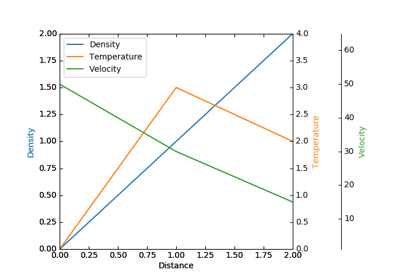Demo Parasite Axes2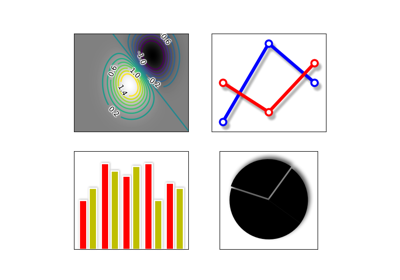Demo Agg Filter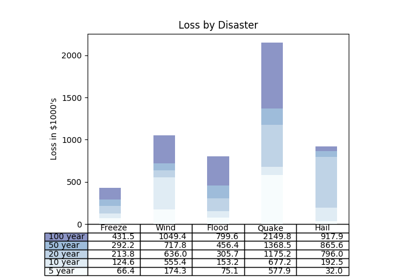Table Demo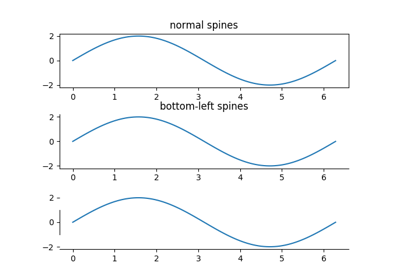Spines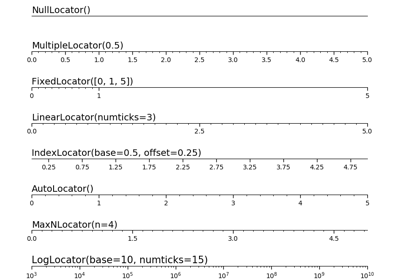Tick locators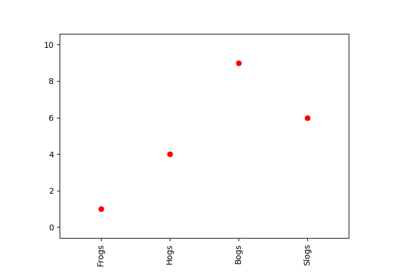Rotating custom tick labels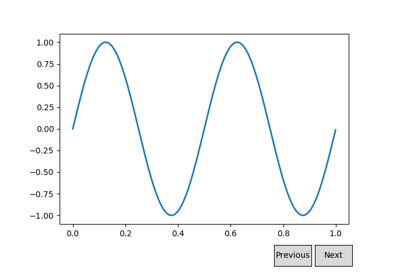Buttons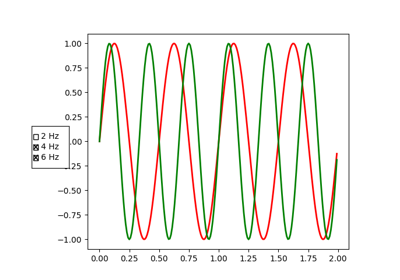Check Buttons